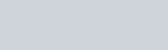for Android
App Name Math Go - Multiplayer Battle v Games, Puzzle10,000+

## Math Go – Multiplayer Battle

Game Math Go – Multiplayer Battle nằm trong danh mục Puzzle

## Giới thiệu Math Go – Multiplayer Battle

Math Go – Multiplayer Battle is a game to test your fast math’s skills.

Learn math’s all operation with fun
– Subtraction
– Multiplication
– Division
– Mixed operations
– Play with friend

Benefits of Using MathGo Or Math Games
• Meets Mathematics Standards
• Easily Linked to Any Mathematics Textbook
• Offers Multiple Assessment Opportunities
• Meets the Needs of Diverse Learners (UA)
• Supports Concept Development in Math
• Encourages Mathematical Reasoning
• Engaging (maintains interest)
• Repeatable (reuse often & sustain involvement
• Open-Ended (allows for multiple approaches & solutions)
• Easy to Prepare
• Easy to Vary for Extended Use & Differentiated Instruction
• Improves Basic Skills
• Enhances Number and Operation Sense
• Encourages Strategic Thinking
• Promotes Mathematical Communication
• Promotes Positive Attitudes Toward Math
• Encourages Parent Involvement

Features
• Addition Games: Simple Addition game to learn math in a fun way.
• Subtraction games: Subtracting numbers to solve the equations.
• Multiplication Games: Multiplication Table is a useful tool for everyone every day.
• Division Games: Enjoy surpassing the division game and learn the division’s table.
• Math games for All: Improve your math skills for various mathematical operations like addition, subtraction, multiplication, and division.
• Brain Training: Here are 10 levels, All levels are suitable for adults and all.
• challenge game: challenge your friend 2 player game.
• Cool math games: Cool Math Games provides all kinds of interesting and fun games for players of all ages and skill levels.
• puzzles in maths: Try using math puzzles to get students strategically thinking about math.
• Educational games: Educational games are games that are designed to help people learn about certain subjects.
• Math learning: its learning games help you to learn cool math examples.
• Math Practice: By doing many basic examples Practices, you will have much impression of all types of examples.
• Math Exercises : Learn Add, Subtract, Multiply & Divide

What is a MathGo?
• The most effective math games are those in which the structure and rules of the games are based on mathematical ideas, and winning a game is directly related to understanding mathematics.
• This game is best for him/her who is looking for “cool math games”
Few bugs fixed regarding leaderboard screen!